# Area of a Rectangle

## How To Find The Area of a Rectangle Using Area of Rectangle Formula.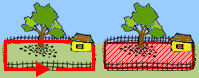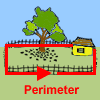The area of a polygon is the number of square units inside the polygon. To understand the difference between perimeter and area, think of perimeter as the length of fence needed to enclose the yard, whereas area is the spaceinside the yard. Perimeter is 1-dimensional and is measured in linear units such as inches, feet or meters. Area is 2-dimensional: it has a length and a width. Area is measured in square units such as square inches, square feet or square meters.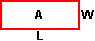To find the area of a rectangle, multiply the length by the width. The formula is:

A = L * W where A is the area, L is the length, W is the width, and * means multiply.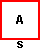square is a rectangle with 4 equal sides. To find the area of a square, multiply the length of one side by itself. The formula is: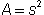orwhere A is the area, s is the length of a side, and · means multiply.

Let's look at some examples of finding the area of rectangles.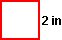Example 1: Find the area of a square with each side measuring 2 inches.

Solution:= (2 in) · (2 in) = 4 in2Example 2: What is the area of a rectangle that has a length of 8 centimeters and a width of 3 centimeters?

Solution: A = L * W

A = (8 cm) · (3 cm) = 24 cm2

In Examples 1 and 2, we found the area of a recentangle given the dimensions of the rectangle. Let's look at some examples in which we are given the area of the rectangle, and are asked to work backwards to find the missing dimension.Example 3: The area of a square is 9 square centimeters. How long is one side?

Solution:9 cm2 =*Since 3 · 3 = 9, we get 3 cm · 3 cm = 9 cm2. Somust equal 3 cm.= 3 cm.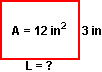Example 4: The area of a rectangle is 12 square inches and the width is 3 inches. What is the length?

Solution: A = L * W

12 in2 = L * 3in

Since 4 · 3 = 12, we get (4 in) · (3 in) = 12 in2. So L must equal 4 in.

L = 4 in.Summary: The dimensions of a rectangle are length and width. Given the length and width of a rectangle, we can find the area. Given the area and one dimension of a rectangle, we can find the other dimension. The formula for area of a rectangle is:

A = L * W  where L is the length and W is the width.A square is a rectangle with 4 equal sides The formula for area of a square is:orwhere S is the length of one side.

### Exercises

Directions: Read each question below. Click once in an ANSWER BOX and type in your answer; then click ENTER. Your answers should be given as whole numbers greater than zero. After you click ENTER, a message will appear in the RESULTS BOX to indicate whether your answer is correct or incorrect. To start over, click CLEAR. This will help you practice finding the area of a rectangle.

 1 Find the area of a square with each side measuring 6 inches. ANSWER BOX:  A =  in2  RESULTS BOX:
 2 A rectangular yard has a length of 12 meters and a width of 4 meters. Find the area. ANSWER BOX:  A =  m2  RESULTS BOX:
 3 The area of a square is 25 square centimeters. How long is one side? ANSWER BOX:=  cm  RESULTS BOX:
 4 The area of a rectangle rug 28 square feet and a length of 7 feet. What is the width? ANSWER BOX:=  ft  RESULTS BOX:
 5 The area of a rectangle paper of 45 square inches and a width of 5 inches. What is the length? ANSWER BOX:=  in  t RESULTS BOX: